CHIRAG GOYAL — May 26, 2021

This article was published as a part of the Data Science Blogathon

## Introduction

Computer Vision is evolving rapidly day-by-day. When we talk about Computer Vision, the term Convolutional Neural Networks (abbreviated as CNN) comes into our mind because CNN is heavily used here.

Therefore it becomes necessary for every aspiring Data Scientist and Machine Learning Engineer to have a good knowledge of these Neural Networks.

In this article, we will discuss the most important questions on the Convolutional Neural Networks(CNNs) which is helpful to get you a clear understanding of the techniques, and also for Data Science Interviews, which covers its very fundamental level to complex concepts.

## 1. What do you mean by Convolutional Neural Network?

A Convolutional neural network (CNN, or ConvNet) is another type of neural network that can be used to enable machines to visualize things.

CNN’s are used to perform analysis on images and visuals. These classes of neural networks can input a multi-channel image and work on it easily with minimal preprocessing required.

These neural networks are widely used in:

• Image recognition and Image classification
• Object detection
• Recognition of faces, etc.

Therefore, CNN takes an image as an input, processes it, and classifies it under certain categories.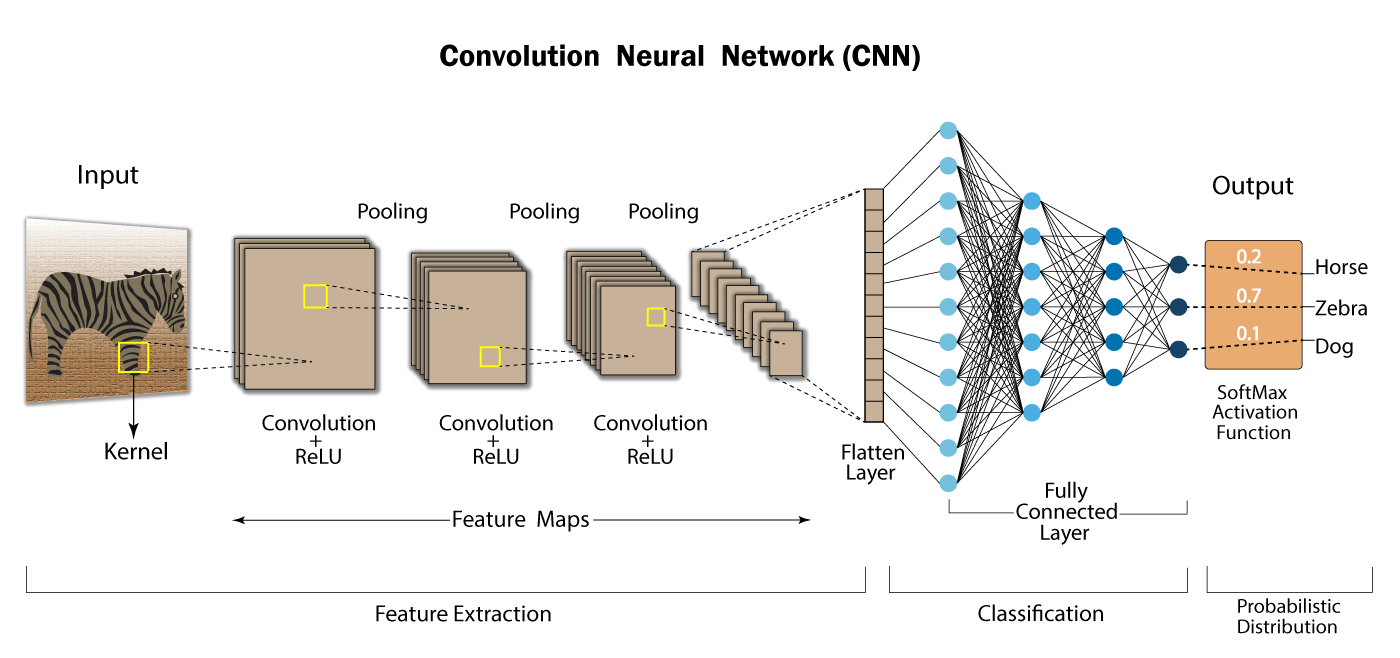## 2. Why do we prefer Convolutional Neural networks (CNN) over Artificial Neural networks (ANN) for image data as input?

1. Feedforward neural networks can learn a single feature representation of the image but in the case of complex images, ANN will fail to give better predictions, this is because it cannot learn pixel dependencies present in the images.

2. CNN can learn multiple layers of feature representations of an image by applying filters, or transformations.

3. In CNN, the number of parameters for the network to learn is significantly lower than the multilayer neural networks since the number of units in the network decreases, therefore reducing the chance of overfitting.

4. Also, CNN considers the context information in the small neighborhood and due to this feature, these are very important to achieve a better prediction in data like images. Since digital images are a bunch of pixels with high values, it makes sense to use CNN to analyze them. CNN decreases their values, which is better for the training phase with less computational power and less information loss.

## 3. Explain the different layers in CNN.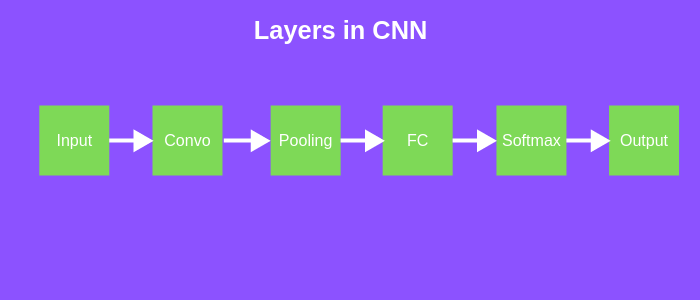The different layers involved in the architecture of CNN are as follows:

1. Input Layer: The input layer in CNN should contain image data. Image data is represented by a three-dimensional matrix. We have to reshape the image into a single column.

For Example, Suppose we have an MNIST dataset and you have an image of dimension 28 x 28 =784, you need to convert it into 784 x 1 before feeding it into the input. If we have “k” training examples in the dataset, then the dimension of input will be (784, k).

2. Convolutional Layer: To perform the convolution operation, this layer is used which creates several smaller picture windows to go over the data.

3. ReLU Layer: This layer introduces the non-linearity to the network and converts all the negative pixels to zero. The final output is a rectified feature map.

4. Pooling Layer: Pooling is a down-sampling operation that reduces the dimensionality of the feature map.

5. Fully Connected Layer: This layer identifies and classifies the objects in the image.

6. Softmax / Logistic Layer: The softmax or Logistic layer is the last layer of CNN. It resides at the end of the FC layer. Logistic is used for binary classification problem statement and softmax is for multi-classification problem statement.

7. Output Layer: This layer contains the label in the form of a one-hot encoded vector.

## 4. Explain the significance of the RELU Activation function in Convolution Neural Network.

RELU Layer – After each convolution operation, the RELU operation is used. Moreover, RELU is a non-linear activation function. This operation is applied to each pixel and replaces all the negative pixel values in the feature map with zero.

Usually, the image is highly non-linear, which means varied pixel values. This is a scenario that is very difficult for an algorithm to make correct predictions. RELU activation function is applied in these cases to decrease the non-linearity and make the job easier.

Therefore this layer helps in the detection of features, decreasing the non-linearity of the image, converting negative pixels to zero which also allows detecting the variations of features.

Therefore non-linearity in convolution(a linear operation) is introduced by using a non-linear activation function like RELU.## 5. Why do we use a Pooling Layer in a CNN?

CNN uses pooling layers to reduce the size of the input image so that it speeds up the computation of the network.

Pooling or spatial pooling layers: Also called subsampling or downsampling.

• It is applied after convolution and RELU operations.
• It reduces the dimensionality of each feature map by retaining the most important information.
• Since the number of hidden layers required to learn the complex relations present in the image would be large.

As a result of pooling, even if the picture were a little tilted, the largest number in a certain region of the feature map would have been recorded and hence, the feature would have been preserved. Also as another benefit, reducing the size by a very significant amount will use less computational power. So, it is also useful for extracting dominant features.

## 6. What is the size of the feature map for a given input size image, Filter Size, Stride, and Padding amount?

Stride tells us about the number of pixels we will jump when we are convolving filters.

If our input image has a size of n x n and filters size f x f and p is the Padding amount and s is the Stride, then the dimension of the feature map is given by:

Dimension = floor[ ((n-f+2p)/s)+1] x floor[ ((n-f+2p)/s)+1]

## 7. An input image has been converted into a matrix of size 12 X 12 along with a filter of size 3 X 3 with a Stride of 1. Determine the size of the convoluted matrix.

To calculate the size of the convoluted matrix, we use the generalized equation, given by:

C = ((n-f+2p)/s)+1

where,

C is the size of the convoluted matrix.

n is the size of the input matrix.

f is the size of the filter matrix.

s is the Stride applied.

Here n = 12, f = 3, p = 0, s = 1

Therefore the size of the convoluted matrix is 10 X 10.

## 8. Explain the terms “Valid Padding” and “Same Padding” in CNN.

Valid Padding: This type is used when there is no requirement for Padding. The output matrix after convolution will have the dimension of (n – f + 1) X (n – f + 1).

Same Padding: Here, we added the Padding elements all around the output matrix. After this type of padding, we will get the dimensions of the input matrix the same as that of the convolved matrix.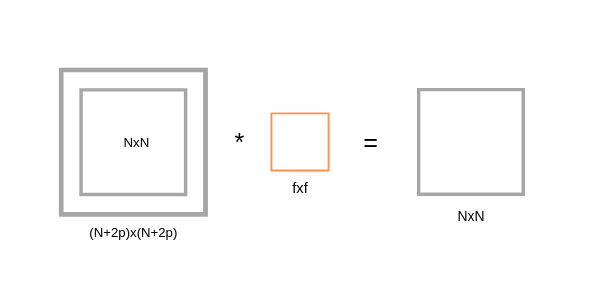After Same padding, if we apply a filter of dimension f x f to (n+2p) x (n+2p) input matrix, then we will get output matrix dimension (n+2p-f+1) x (n+2p-f+1). As we know that after applying Padding we will get the same dimension as the original input dimension (n x n). Hence we have,

(n+2p-f+1)x(n+2p-f+1) equivalent to nxn

n+2p-f+1 = n

p = (f-1)/2

So, by using Padding in this way we don’t lose a lot of information and the image also does not shrink.

## 9. What are the different types of Pooling? Explain their characteristics.

Spatial Pooling can be of different types –  max pooling, average pooling, and Sum pooling.

• Max pooling: Once we obtain the feature map of the input, we will apply a filter of determined shapes across the feature map to get the maximum value from that portion of the feature map. It is also known as subsampling because from the entire portion of the feature map covered by filter or kernel we are sampling one single maximum value.
• Average pooling: Computes the average value of the feature map covered by kernel or filter, and takes the floor value of the result.
• Sum pooling: Computes the sum of all elements in that window.

Characteristics:

Max pooling returns the maximum value of the portion covered by the kernel and suppresses the Noise, while Average pooling only returns the measure of that portion.

The most widely used pooling technique is max pooling since it captures the features of maximum importance with it.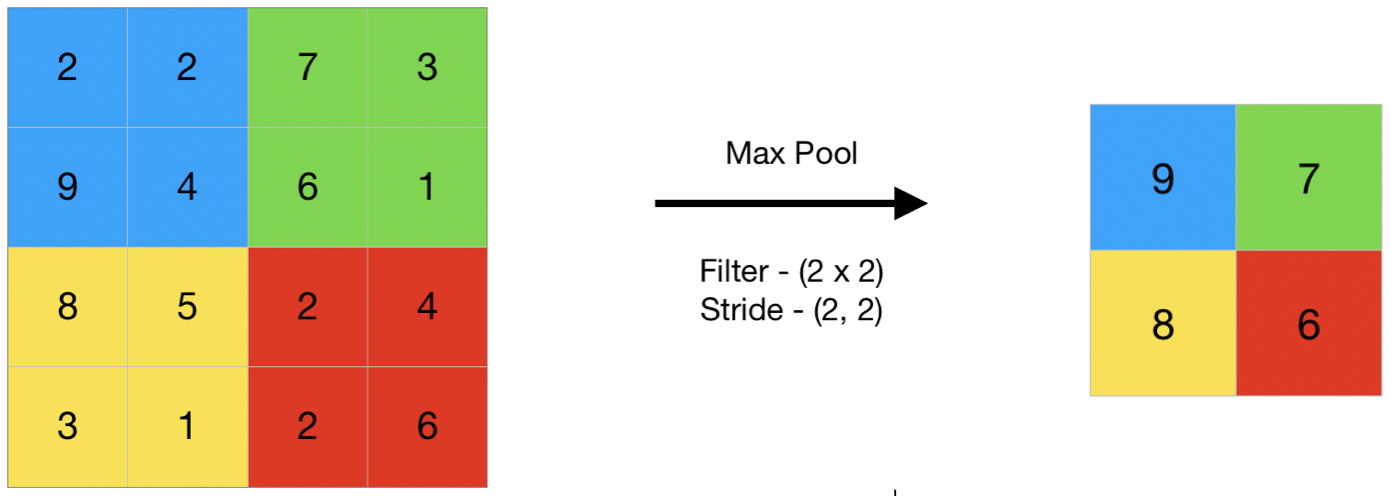## 10. Does the size of the feature map always reduce upon applying the filters? Explain why or why not.

No, the convolution operation shrinks the matrix of pixels(input image) only if the size of the filter is greater than 1 i.e, f > 1.

When we apply a filter of 1×1, then there is no reduction in the size of the image and hence there is no loss of information.

## 11. What is Stride? What is the effect of high Stride on the feature map?

Stride refers to the number of pixels by which we slide over the filter matrix over the input matrix. For instance –

• If Stride =1, then move the filter one pixel at a time.
• If Stride=2, then move the filter two-pixel at a time.

Moreover, larger Strides will produce a smaller feature map.

## 12. Explain the role of the flattening layer in CNN.

After a series of convolution and pooling operations on the feature representation of the image, we then flatten the output of the final pooling layers into a single long continuous linear array or a vector.

The process of converting all the resultant 2-d arrays into a vector is called Flattening.

Flatten output is fed as input to the fully connected neural network having varying numbers of hidden layers to learn the non-linear complexities present with the feature representation.

## 13. List down the hyperparameters of a Pooling Layer.

The hyperparameters for a pooling layer are:

• Filter size
• Stride
• Max or average pooling

If the input of the pooling layer is nh x nw x nc, then the output will be –

Dimension = [ {(nh – f) / s + 1}* {(nw – f) / s + 1}* nc’ ]

## 14. What is the role of the Fully Connected (FC) Layer in CNN?

The aim of the Fully connected layer is to use the high-level feature of the input image produced by convolutional and pooling layers for classifying the input image into various classes based on the training dataset.

Fully connected means that every neuron in the previous layer is connected to each and every neuron in the next layer. The Sum of output probabilities from the Fully connected layer is 1, fully connected using a softmax activation function in the output layer.

The softmax function takes a vector of arbitrary real-valued scores and transforms it into a vector of values between 0 and 1 that sums to 1.

Working

It works like an ANN, assigning random weights to each synapse, the input layer is weight-adjusted and put into an activation function. The output of this is then compared to the true values and the error generated is back-propagated, i.e. the weights are re-calculated and repeat all the processes. This is done until the error or cost function is minimized.

## 15. Briefly explain the two major steps of CNN i.e, Feature Learning and Classification.

Feature Learning deals with the algorithm by learning about the dataset. Components like Convolution, ReLU, and Pooling work for that, with numerous iterations between them. Once the features are known, then classification happens using the Flattening and Full Connection components.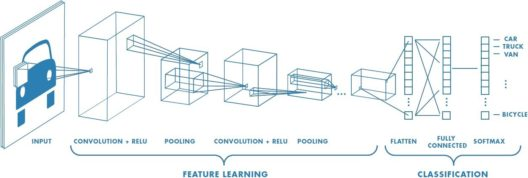## 16. What are the problems associated with the Convolution operation and how can one resolve them?

As we know, convolving an input of dimensions 6 X 6 with a filter of dimension 3 X 3 results in the output of 4 X 4 dimension. Let’s generalize the idea:

We can generalize it and say that if the input is n X n and the Filter Size is f X f, then the output size will be (n-f+1) X (n-f+1):

• Input: n X n
• Filter size: f X f
• Output: (n-f+1) X (n-f+1)

There are primarily two disadvantages here:

• When we apply a convolutional operation, the size of the image shrinks every time.
• Pixels present in the corner of the image i.e, in the edges, are used only a few times during convolution as compared to the central pixels. Hence, we do not focus too much on the corners so it can lead to information loss.

To overcome these problems, we can apply the padding to the images with an additional border, i.e., we add one pixel all around the edges. This means that the input will be of the dimension 8 X 8 instead of a 6 X 6 matrix. Applying convolution on the input of filter size 3 X 3 on it will result in a 6 X 6 matrix which is the same as the original shape of the image. This is where Padding comes into the picture:

Padding: In convolution, the operation reduces the size of the image i.e, spatial dimension decreases thereby leading to information loss. As we keep applying convolutional layers, the size of the volume or feature map will decrease faster.

Zero Paddings allow us to control the size of the feature map.

Padding is used to make the output size the same as the input size.

Padding amount = number of rows and columns that we will insert in the top, bottom, left, and right of the image. After applying padding,

• Input: n X n
• Filter size: f X f
• Output: (n+2p-f+1) X (n+2p-f+1)

## 17. Let us consider a Convolutional Neural Network having three different convolutional layers in its architecture as –

Layer-1: Filter Size – 3 X 3, Number of Filters – 10, Stride – 1, Padding – 0

Layer-2: Filter Size – 5 X 5, Number of Filters – 20, Stride – 2, Padding – 0

Layer-3: Filter Size – 5 X5 , Number of Filters – 40, Stride – 2, Padding – 0

### If we give the input a 3-D image to the network of dimension 39 X39, then determine the dimension of the vector after passing through a fully connected layer in the architecture.

Here we have the input image of dimension 39 X 39 X 3 convolves with 10 filters of size 3 X 3 and takes the Stride as 1 with no padding. After these operations, we will get an output of 37 X 37 X 10.

We then convolve this output further to the next convolution layer as an input and get an output of 7 X 7 X 40. Finally, by taking all these numbers (7 X 7 X 40 = 1960), and then unroll them into a large vector, and pass them to a classifier that will make predictions.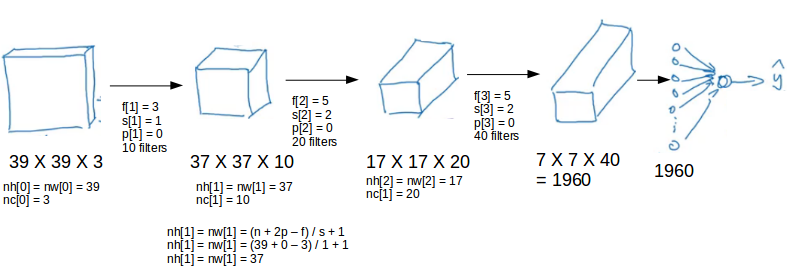## 18. Explain the significance of “Parameter Sharing” and “Sparsity of connections” in CNN.

Parameter sharing: In convolutions, we share the parameters while convolving through the input. The intuition behind this is that a feature detector, which is useful in one part of the image may also be useful in another part of the image. So, by using a single filter we convolved all the entire input and hence the parameters are shared.

Let’s understand this with an example,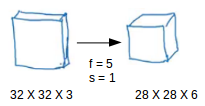If we would have used just the fully connected layer, the number of parameters would be = 32*32*3*28*28*6, which is nearly equal to 14 million which makes no sense.

But in the case of a convolutional layer, the number of parameters will be = (5*5 + 1) * 6 (if there are 6 filters), which is equal to 156. Convolutional layers, therefore, reduce the number of parameters and speed up the training of the model significantly.

The sparsity of Connections: This implies that for each layer, each output value depends on a small number of inputs, instead of taking into account all the inputs.

## 19. Explain the role of the Convolution Layer in CNN.

Convolution is a linear operation of a smaller filter to a larger input that results in an output feature map.

Convolution layer: This layer performs an operation called a convolution, hence the network is called a convolutional neural network. It extracts features from the input images. Convolution is a linear operation that involves the multiplication of a set of weights with the input.

This technique was designed for 2d-input(array of data). The multiplication is performed between an array of input data and a 2d array of weights called a filter or kernel.

This is the component that detects features in images preserving the relationship between pixels by learning image features using small squares of input data i.e, respecting their spatial boundaries.

## 20. Can we use CNN to perform Dimensionality Reduction? If Yes then which layer is responsible for dimensionality reduction particularly in CNN?

Yes, CNN does perform dimensionality reduction. A pooling layer is used for this.

The main objective of Pooling is to reduce the spatial dimensions of a CNN. To reduce the spatial dimensionality, it will perform the down-sampling operations and creates a pooled feature map by sliding a filter matrix over the input matrix.

## Discussion Problem

Let us consider a Convolutional Neural Network having two different Convolutional Layers in the Architecture i.e,

Layer-1: Filter Size: 5 X 5, Number of Filters: 6, Stride-1, Padding-0, Max-Pooling: (Filter Size: 2 X 2 with Stride-2)

Layer-2: Filter Size: 5 X 5, Number of Filters: 16, Stride-1, Padding-0, Max-Pooling: (Filter Size: 2 X 2 with Stride-2)

If we give a 3-D image as the input to the network of dimension 32 X 32, then the dimension of the vector after passing through a flattening layer in the architecture is _____?

## End Notes

I hope you enjoyed the questions and were able to test your knowledge about Convolutional Neural Networks.

If you liked this and want to know more, go visit my other articles on Data Science and Machine Learning by clicking on the Link

Something not mentioned or want to share your thoughts? Feel free to comment below And I’ll get back to you.